# Multiplexer

Multiplexer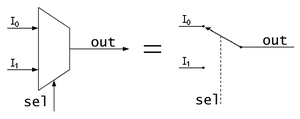Schematic of a 2-to-1 Multiplexer. It can be equated to a controlled switch.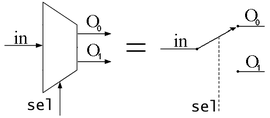Schematic of a 1-to-2 Demultiplexer. Like a multiplexer, it can be equated to a controlled switch.

In electronics, a multiplexer (or mux) is a device that selects one of several analog or digital input signals and forwards the selected input into a single line. A multiplexer of 2n inputs has n select lines, which are used to select which input line to send to the output. Multiplexers are mainly used to increase the amount of data that can be sent over the network within a certain amount of time and bandwidth. A multiplexer is also called a data selector.

An electronic multiplexer makes it possible for several signals to share one device or resource, for example one A/D converter or one communication line, instead of having one device per input signal.

On the other hand, a demultiplexer (or demux) is a device taking a single input signal and selecting one of many data-output-lines, which is connected to the single input. A multiplexer is often used with a complementary demultiplexer on the receiving end.

An electronic multiplexer can be considered as a multiple-input, single-output switch, and a demultiplexer as a single-input, multiple-output switch. The schematic symbol for a multiplexer is an isosceles trapezoid with the longer parallel side containing the input pins and the short parallel side containing the output pin. The schematic on the right shows a 2-to-1 multiplexer on the left and an equivalent switch on the right. The sel wire connects the desired input to the output.

## Telecommunications

In telecommunications, a multiplexer is a device that combines several input information signals into one output signal, which carries several communication channels, by means of some multiplex technique. A demultiplexer is, in this context, a device taking a single input signal that carries many channels and separates those over multiple output signals.

Multiplex
techniques
Circuit mode
(constant bandwidth)
TDM · FDM · SDM
Polarization multiplexing
Spatial multiplexing (MIMO)
Statistical multiplexing
(variable bandwidth)
Packet mode · Dynamic TDM
FHSS · DSSS
OFDMA · SC-FDM · MC-SS
Related topics
Channel access methods
Media Access Control (MAC)

This box:

In telecommunications and signal processing, an analog time division multiplexer (TDM) may take several samples of separate analogue signals and combine them into one pulse amplitude modulated (PAM) wide-band analogue signal. Alternatively, a digital TDM multiplexer may combine a limited number of constant bit rate digital data streams into one data stream of a higher data rate, by forming data frames consisting of one timeslot per channel.

In telecommunications, computer networks and digital video, a statistical multiplexer may combine several variable bit rate data streams into one constant bandwidth signal, for example by means of packet mode communication. An inverse multiplexer may utilize several communication channels for transferring one signal.

## Cost savings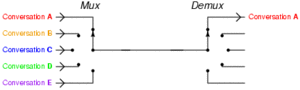The basic function of a multiplexer: combining multiple inputs into a single data stream. On the receiving side, a demultiplexer splits the single data stream into the original multiple signals.

One use for multiplexers is cost savings by connecting a multiplexer and a demultiplexer (or demux) together over a single channel (by connecting the multiplexer's single output to the demultiplexer's single input). The image to the right demonstrates this. In this case, the cost of implementing separate channels for each data source is more expensive than the cost and inconvenience of providing the multiplexing/demultiplexing functions. In a physical analogy, consider the merging behaviour of commuters crossing a narrow bridge; vehicles will take turns using the few available lanes. Upon reaching the end of the bridge they will separate into separate routes to their destinations.

At the receiving end of the data link a complementary demultiplexer is normally required to break single data stream back down into the original streams. In some cases, the far end system may have more functionality than a simple demultiplexer and so, while the demultiplexing still exists logically, it may never actually happen physically. This would be typical where a multiplexer serves a number of IP network users and then feeds directly into a router which immediately reads the content of the entire link into its routing processor and then does the demultiplexing in memory from where it will be converted directly into IP packets.

Often, a multiplexer and demultiplexer are combined together into a single piece of equipment, which is usually referred to simply as a "multiplexer". Both pieces of equipment are needed at both ends of a transmission link because most communications systems transmit in both directions.

A real world example is the creation of telemetry for transmission from the computer/instrumentation system of a satellite, space craft or other remote vehicle to a ground-based system.

In analog circuit design, a multiplexer is a special type of analog switch that connects one signal selected from several inputs to a single output.

## Digital multiplexers

In digital circuit design, the selector wires are of digital value. In the case of a 2-to-1 multiplexer, a logic value of 0 would connect$I_0$ to the output while a logic value of 1 would connect$I_1$ to the output. In larger multiplexers, the number of selector pins is equal to$\left \lceil \log_2(n) \right \rceil$ where$n$ is the number of inputs.

For example, 9 to 16 inputs would require no fewer than 4 selector pins and 17 to 32 inputs would require no fewer than 5 selector pins. The binary value expressed on these selector pins determines the selected input pin.

A 2-to-1 multiplexer has a boolean equation where$A$ and$B$ are the two inputs,$S$ is the selector input, and$Z$ is the output:$Z = ( A \cdot \overline{S}) + (B \cdot S)$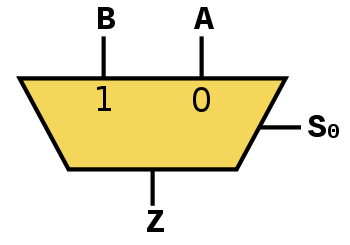A 2-to-1 mux

Which can be expressed as a truth table:$S$$A$$B$$Z$ 0 1 1 1 1 0 1 0 1 0 0 0 0 1 1 1 1 1 0 0 0 1 1 0 0 0

This truth table shows that when$S=0$ then$Z=A$ but when$S=1$ then$Z=B$. A straightforward realization of this 2-to-1 multiplexer would need 2 AND gates, an OR gate, and a NOT gate.

Larger multiplexers are also common and, as stated above, require$\left \lceil \log_2(n) \right \rceil$ selector pins for n inputs. Other common sizes are 4-to-1, 8-to-1, and 16-to-1. Since digital logic uses binary values, powers of 2 are used (4, 8, 16) to maximally control a number of inputs for the given number of selector inputs.

The boolean equation for a 4-to-1 multiplexer is:$F = (A \cdot \overline{S_0} \cdot \overline{S_1}) + (B \cdot S_0 \cdot \overline {S_1}) + (C \cdot \overline{S_0} \cdot S_1 ) + (D \cdot S_0 \cdot S_1)$

Two realizations for creating a 4-to-1 multiplexer are shown below: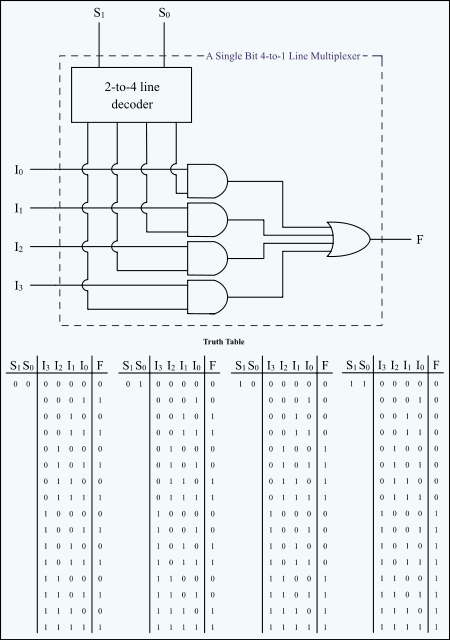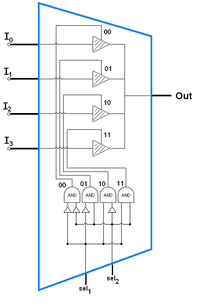These are two realizations of a 4-to-1 multiplexer: one realized from a decoder, AND gates, and an OR gate one realized from 3-state buffers and AND gates (the AND gates are acting as the decoder) Note that the subscripts on the$I_n$ inputs indicate the decimal value of the binary control inputs at which that input is let through.

### Chaining multiplexers

Larger multiplexers can be constructed by using smaller multiplexers by chaining them together. For example, an 8-to-1 multiplexer can be made with two 4-to-1 and one 2-to-1 multiplexers. The two 4-to-1 multiplexer outputs are fed into the 2-to-1 with the selector pins on the 4-to-1's put in parallel giving a total number of selector inputs to 3, which is equivalent to an 8-to-1.

### List of ICs which provide multiplexing

The 7400 series has several ICs that contain multiplexer(s):

S.No. IC No. Function Output State
1 74157 Quad 2:1 mux. Output same as input given
2 74158 Quad 2:1 mux. Output is inverted input
3 74153 Dual 4:1 mux. Output same as input
4 74352 Dual 4:1 mux. Output is inverted input
5 74151A 8:1 mux. Both outputs available (i.e., complementary outputs)
6 74151 8:1 mux. Output is inverted input
7 74150 16:1 mux. Output is inverted input

## Digital demultiplexers

Demultiplexers take one data input and a number of selection inputs, and they have several outputs. They forward the data input to one of the outputs depending on the values of the selection inputs. Demultiplexers are sometimes convenient for designing general purpose logic, because if the demultiplexer's input is always true, the demultiplexer acts as a decoder. This means that any function of the selection bits can be constructed by logically OR-ing the correct set of outputs.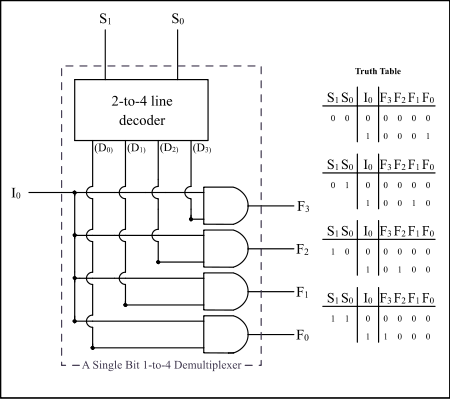Example: A Single Bit 1-to-4 Line Demultiplexer

### List of ICs which provide demultiplexing

The 7400 series has several ICs that contain demultiplexer(s):

S.No. IC No. (7400) IC No. (4000) Function Output State
1 74139 Dual 1:4 demux. Output is inverted input
3 74156 Dual 1:4 demux. Output is open collector
4 74138 1:8 demux. Output is inverted input
5 74238 1:8 demux. Output is same as input
6 74154 1:16 demux. Output is inverted input
7 74159 CD4514/15 1:16 demux. Output is open collector and same as input

## Multiplexers as PLDs

Multiplexers can also be used as programmable logic devices. By specifying the logic arrangement in the input signals, a custom logic circuit can be created. The selector inputs then act as the logic inputs. This is especially useful in situations when cost is a factor and for modularity.

Wikimedia Foundation. 2010.

Поможем написать реферат

### Look at other dictionaries:

• multiplexer — mul‧ti‧plex‧er [ˈmʌltɪˌpleksə ǁ ər] noun [countable] COMPUTING a piece of equipment used to send several electrical signals using only one connection, used especially with modem S * * * multiplexer UK US /ˈmʌltiˌpleksər/ noun [C] IT ► a piece of …   Financial and business terms

• multiplexer — ● multiplexer verbe transitif Effectuer un multiplexage …   Encyclopédie Universelle

• multiplexer — /multiplɛkser, ingl. ˈmʌltɪpl0ksə(r)/ [vc. ingl., da multiplex «di più parti»] s. m. inv. (tel.) multiplatore, multiplex …   Sinonimi e Contrari. Terza edizione

• Multiplexer — Animation des Prinzips eines Multiplexers Ein Multiplexer (kurz: MUX) ist ein Selektionsschaltnetz in der analogen Elektronik und Digitaltechnik, mit dem aus einer Anzahl von Eingangssignalen eines ausgewählt werden kann, etwa bei einem… …   Deutsch Wikipedia

• Multiplexer — I Multiplexer,   ein Gerät, das gleichzeitig eintreffende Datenströme so umwandelt oder anordnet, dass sie praktisch gleichzeitig auf einem einzigen Datenübertragungskanal übertragen werden können. Man unterscheidet Frequenzmultiplexer, die den… …   Universal-Lexikon

• Multiplexer — multiplekseris statusas T sritis automatika atitikmenys: angl. multiplexer vok. Multiplexer, m rus. мультиплексор, m pranc. multiplexeur, m ryšiai: sinonimas – tankintuvas …   Automatikos terminų žodynas

• multiplexer — multiplekseris statusas T sritis automatika atitikmenys: angl. multiplexer vok. Multiplexer, m rus. мультиплексор, m pranc. multiplexeur, m ryšiai: sinonimas – tankintuvas …   Automatikos terminų žodynas

• Multiplexer — Mul|ti|ple|xer* der; s, <aus gleichbed. engl. multiplexer; vgl. ↑multiplex> Gerät, das Signale von mehreren Eingangsleitungen auf eine Ausgangsleitung überträgt (EDV) …   Das große Fremdwörterbuch

• multiplexer —    Often abbreviated mux. A device that merges several lower speed transmissionchannelsintoonehigh speedchannel at one end of the link. Another multiplexer reverses this process at the other end of the link to reproduce the low speed channels.… …   Dictionary of networking

• multiplexer — tankintuvas statusas T sritis informatika apibrėžtis Telekomunikacijose – įtaisas, sujungiantis keletą skirtingų duomenų ↑ kanalų (srautų) į vieną kanalą. Naudojamas, kai reikia daug kanalų (ryšio linijų) prijungti prie mažesnio prievadų… …   Enciklopedinis kompiuterijos žodynas